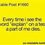# On the Question on Arrays

Previously, I posted a question on arrays and asked for a solution.

$\begin{bmatrix} 2&0&1&0&2&0\\ 0&2&0&1&2&0\\ 1&0&2&0&2&0\\ 0&1&0&2&2&0\\ 1&1&1&1&2&0\\ 0&0&0&0&0&0 \end{bmatrix}$ In the above $$6 \times 6$$ array, one can choose any $$k \times k$$ subarray, with $$1 < k \leq 6$$ and add $$1$$ to all its entries. Is it possible to perform the operation a finite number of times such that all entries in the array are multiples of 3?

This was because I did not understand the solution given. This was the answer from the Singapore Mathematical Olympiad 2013 ( Senior )

The answer is no. Let the original array be $A$. Consider the following array $M= \begin{bmatrix} 0&1&1&-1&-1&0\\ -1&0&1&-1&0&1\\ -1&-1&0&0&1&1\\ 1&1&0&0&-1&-1\\ 1&0&-1&1&0&-1\\ 0&-1&-1&1&1&0 \end{bmatrix}.$ Multiply the corresponding elements of the two arrays and compute the sum modulo 3. It's easy to verify that this sum is invariant under the given operation. Since the original sum is 2, one can never obtain an array where all the entries are multiples of 3.

What does this mean? I think that the answer given is pretty vague, and I do not understand the reason or purpose of multiplying the elements of $A$ and $M$, or even how M was derived in the first place. What does this have to do with the original operation in the question?Note by Timothy Wan
5 years, 1 month ago

This discussion board is a place to discuss our Daily Challenges and the math and science related to those challenges. Explanations are more than just a solution — they should explain the steps and thinking strategies that you used to obtain the solution. Comments should further the discussion of math and science.

When posting on Brilliant:

• Use the emojis to react to an explanation, whether you're congratulating a job well done , or just really confused .
• Ask specific questions about the challenge or the steps in somebody's explanation. Well-posed questions can add a lot to the discussion, but posting "I don't understand!" doesn't help anyone.
• Try to contribute something new to the discussion, whether it is an extension, generalization or other idea related to the challenge.

MarkdownAppears as
*italics* or _italics_ italics
**bold** or __bold__ bold
- bulleted- list
• bulleted
• list
1. numbered2. list
1. numbered
2. list
Note: you must add a full line of space before and after lists for them to show up correctly
paragraph 1paragraph 2

paragraph 1

paragraph 2

[example link](https://brilliant.org)example link
> This is a quote
This is a quote
    # I indented these lines
# 4 spaces, and now they show
# up as a code block.

print "hello world"
# I indented these lines
# 4 spaces, and now they show
# up as a code block.

print "hello world"
MathAppears as
Remember to wrap math in $$ ... $$ or $ ... $ to ensure proper formatting.
2 \times 3 $2 \times 3$
2^{34} $2^{34}$
a_{i-1} $a_{i-1}$
\frac{2}{3} $\frac{2}{3}$
\sqrt{2} $\sqrt{2}$
\sum_{i=1}^3 $\sum_{i=1}^3$
\sin \theta $\sin \theta$
\boxed{123} $\boxed{123}$

Sort by:

The idea is that you want to find a property that is invariant under the allowed operations. Then, if can calculate the value of the property for matrix A, and see how that compares with the value of the property for "all entries are multiples of 3".

Calculating the sum modulo 3 makes a lot of sense since we want them to be multiples of 3. Other possibilities could be modulo 6 or 9.

The "multiply the corresponding elements" simply means "weight the elements by this extent" IE the contribution of the first row of A is equal to $2 \times 0 + 0 \times 1 + 1 \times 1 + 0 \times -1 + 2 \times -1 + 0 \times 0$.

Coming up with the matrix M is much harder. It is not immediately apparent why that would work. It takes some practice and ideas to figure out how to approach the problem.

Staff - 5 years, 1 month ago